## Simple Populations: n=6, l=8

Everybody can try to play around with different settings. Here only two more examples demonstrating the truth of our considerations above with regard to the probabilities. The main point is, that a population is not stable with regard to it's overall fitness value. As long as mutation can operate there is a momentum of randomness which takes it's course along the highest probabilities; but exactly these are changing depending from the history so far (all examples are computed with the GA_v4.sce program; see section with 'Programs').

The following examples - out of many more - are taken from the setting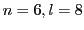. The first example (cf. figure 5.8) starts with a random population generated with the function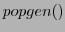, and the second example (cf. figure 5.9) starts with a maximal population created by the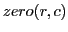function from scilab.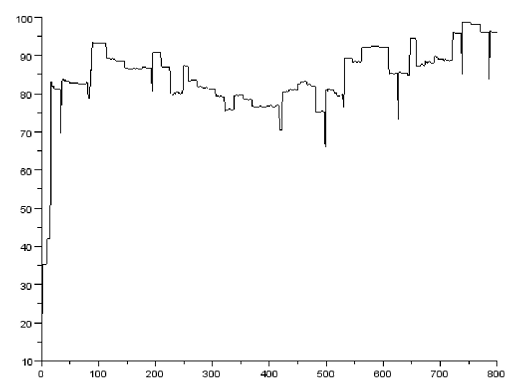In the first example with the random population with a low overall fitness value at the beginning the probability is very high to improve. This can be seen from the diagram 5.8. After reaching quite the mximum the probability is increased to weaken again. Within the scope of the 800 cycles this didn't happen too much.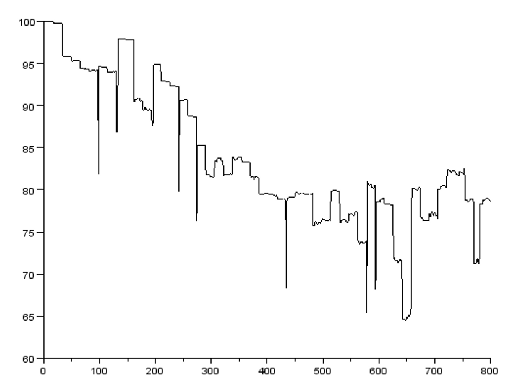In the second example a population with a maximal overall fitness value has been generated at will.

```-->POPmax=ones(6,8)
POPmax  =

1.    1.    1.    1.    1.    1.    1.    1.
1.    1.    1.    1.    1.    1.    1.    1.
1.    1.    1.    1.    1.    1.    1.    1.
1.    1.    1.    1.    1.    1.    1.    1.
1.    1.    1.    1.    1.    1.    1.    1.
1.    1.    1.    1.    1.    1.    1.    1.
```

In this population there is a high probability from the beginning to degrade. As the diagram 5.9 demonstrates nicely this did happen in one example.

Gerd Doeben-Henisch 2013-01-14